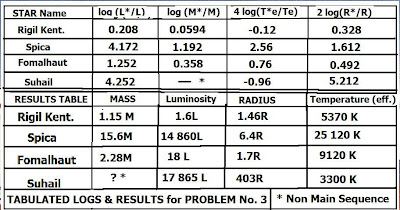## Thursday, September 29, 2011

### Solutions to Intermediate Astronomy (9)The Problems recapped again, with solutions:

1) Find the radius of the star Alhena from Problem (1) in the previous problem set. (Use the same data available)

Solution:

To obtain the radius we use:

2 log (R*/R) = log (L*/L) - 4 log (T_eff*/T)

Where (from the data in previous problem (1):

log (L*/L) = 0.4 (4.63 - (-1.18)) = 0.4 (5.81) = 2.324

SO:

2 log (R*/R) = 2.324 - 4(4.03 - 3.76) = 1.244

Or:

log (R*/R) = 1.244/2 = 0.622 and antilog (0.622) = 4.2

Thus: (R*/R) = 4.2 and R* = 4.2 R or 4.2x the radius of the Sun.

2) Use two different techniques to arrive at the radius of the star Beta Pegasi, which lies at a distance of 50 pc and has: (B - V) = +1.7 and m_v = 2.5. Interferometry obtains the apparent angular diameter as 0."021.

a) What is the apparent discrepancy (as a percentage) in the two values obtained?

b) Account for these discrepancies.

c) Which value, if any, would you be inclined to assign as having greater accuracy?

Solutions:

Begin by converting to AU:

50 pc = 50 pc x (2 x 10^5 AU/pc) = 10^7 AU

We use the relation:

R*/R = (d* a*")/ (d a")

where: d* = 10^7 AU, a*" = 0."021

d = 1 AU, and a" = 1920"

Then:

R*/R = (0."021/ 1920") x (10^7 ) = 109.3

Thus, by interferometry, R* = 109.3 R

Or, Beta Pegasi is 109.3 times the radius of the Sun.

By photometry:

(B - V) = +1.7 so B.C. = -1.7, while m_v = +2.5

M_V = m_v - 5 log d + 5 = 2.5 - 5 log (40) + 5 = 2.5 - 8.5 + 5 = -1

M_bol = M_V + B.C. = -1 + (-1.7) = -2.7

Log (L*/L) = 0.4 (M_bol - M_bol*) = 0.4(4.63 - (-2.7)) = 0.4(7.33) = 2.93

2 log (R*/R) = log (L*/L) - 4 log (T_eff*/T)

Using the table of Bolometric corrections vs. T_eff we find for B.C. = -1.7:

log (T_eff) = 3.52

And we know log (T) = log (5760) = 3.76

So: 2 log (R*/R) = 2.93 - 4(3.52 - 3.76) = 2.93 + 0.96 = 3.89

log (R*/R) = 3.89/ 2 = 1.946

and: R*/R = 88.3 (Since antilog (1.946) = 88.3)

Now:

(a) The apparent discrepancy is the difference in the results:

% diff. = [(109.4 R = 88.3R)/ 109.4 R] x 100% = 19% approx.

(b) Both values suffer from about the same degree of uncertainty, so that a mean value, e.g.:

R_av = ½(109. 4 + 88.3)R

would be the best.

c) The interferometry method has a significant probable error owing to the fact that Beta Pegasi is at the very limit of practical application for the trigonometric parallax distance technique (~ 50 pc). A similar uncertainty also appears in the photometric method, e.g. in the determination of M_V and henc also M_bol, log (L*/L) and R*/R. Thus, there is no appreciable benefit in selecting one method over the other.

3) Consider the stars below and their (B- V) and M_V values:

Rigil Kent : (B - V) = 0.71, M_V = 4.2

Spica: (B - V) = -0.23, and M_V = (-3.1)

Fomalhaut: (B - V) = 0.09 and M_V = 1.9

Suhail: (B - V) = 1.7 and M_V = (-4.3)

a) Find the mass, luminosity and radius of each of these stars.

b) Try to estimate the surface temperature of each of these stars. (Hint: you can use the data from the Table of Bolometric corrections in the previous instalment).

Solutions:

The most expeditious way to solve for this set is to first prepare a calculation table based on the key logs for the assorted relationships. These are: log (M*/M), log (L*/L), 4 log (T*_eff/T_eff) and 2 log (R*/R). The method of obtaining each of the preceding is simply based on computations we've already shown, given the data indicated, e.g. (B - V), M_V. The upper part of the Table presented shows the assorted log computation results. Note that Suhail doesn't have a proper mass value, since the mass-luminosity index isn't valid being off the main sequence.

The lower region of the table shows the assorted mass, luminosity, radius values based on the preceding log table. (E.g. we work out the radius, using the given log results for temperature and luminosity, such as demonstrated in Problems 1,2. Again, Suhail's mass is in question. Note, we can stil obtain a radius and luminosity for it by relying exclusively on the (B - V) and M_V values and making reference to an H-R (Hertzprung -Russell ) diagram to get estimates.

(b) Surface temperatures can be obtained using the Bolometric corrections table (Instalment #8), and referencing the (B - V) to the appropriate log (T_eff). Thus, for Rigil Kent, (B - V) = 0.71 which corresponds to log (T_eff) = 3.73 from the Table. Then taking the antilog, we obtain T_eff = 5370 K. In the case of Spica, (B - V) = -0.23 and we see tabulated values given in the table for -0.20 (4.32) and -0.25 (log T_eff = 4.46), so we interpolate the value for -0.23 (e.g. 3/5 of the scale between -0.20 and -0.25) to get log T_eff = 4.40 or T_eff = 25 120 approx.